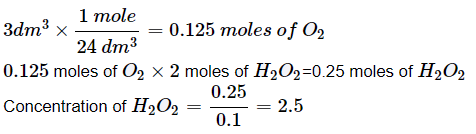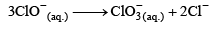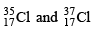Courses

# Test: Mole Concept, Volumetric & Redox- 2

## 30 Questions MCQ Test Physical Chemistry | Test: Mole Concept, Volumetric & Redox- 2

Description
This mock test of Test: Mole Concept, Volumetric & Redox- 2 for Chemistry helps you for every Chemistry entrance exam. This contains 30 Multiple Choice Questions for Chemistry Test: Mole Concept, Volumetric & Redox- 2 (mcq) to study with solutions a complete question bank. The solved questions answers in this Test: Mole Concept, Volumetric & Redox- 2 quiz give you a good mix of easy questions and tough questions. Chemistry students definitely take this Test: Mole Concept, Volumetric & Redox- 2 exercise for a better result in the exam. You can find other Test: Mole Concept, Volumetric & Redox- 2 extra questions, long questions & short questions for Chemistry on EduRev as well by searching above.
QUESTION: 1

Solution:
QUESTION: 2

Solution:
QUESTION: 3

### 0.3g of an oxalate salt was dissolved in 100 ml solution. The solution required 90 ml of N/20 KMnO4 for complete oxidation. The % of oxalateion in salt is:

Solution:
QUESTION: 4

The number of moles of KMnO4 that will be needed to react with one mole of sulphite ion in acidic solution is:

Solution:
QUESTION: 5

A certain compound has the molecular formula X4O6. If 10g of compound contains 5.62g of x, the atomic mass of x is approx:

Solution:
QUESTION: 6

Starch paper is used to test for the presence of [NCERT 1979]

Solution:
QUESTION: 7

When a solution of NaOH and Na2CO3 is titrated against standard HCl, the end point due to phenolphthalein is obtained after the reaction:

Solution:
QUESTION: 8

Cu2+ + I → Cu+ + I2, I2 + Na2S2O3 → NaI + Na2S4O6

If the moles of Cu2+ in above reaction consumed is ‘a’ then equivalents of Na2S4O6 will be:

Solution:
QUESTION: 9

In the above equation if moles required of S2O32– to titrate I2 was 2 then what were the moles of IO3 used:

IO3 + I + H+ → I2 + H2O, I2 + S2O32– → I + S4O62–

Solution:
QUESTION: 10

Then factor Of FeS2 in the following reaction  FeS2 → Fe3+ + SO2 would be:

Solution:
QUESTION: 11

Fe0.94O → Fe3+, equivalent weight of reactants will be:

Solution:
QUESTION: 12

H3PO4 is a tribasic acid and one of its salt is NaH2PO4 . What volume of 1M NaOH solution should be added to 12g of NaH2PO4 to convert it into Na3PO4:

Solution:
QUESTION: 13

Sulphuryl chloride SO2Cl2, reacts with H2O to give mixture of H2SO4 and HCl. Aqueous solution of 1mole of SO2Cl2 will be neutralized by:

Solution:
QUESTION: 14

When Cr2O72– ion act as an oxidant in acidic medium, Cr3+ ion is formed. The number of moles of Sn2+ that are oxidized to Sn4+ by one mole of Cr2O72– ion would be:

Solution:
QUESTION: 15

A 0.518g sample of limestone is dissolved in HCl and then calcium is precipitated as CaC2O4. After filtering and washing the precipitate it requires 60 ml of 0.25N KMnO4 solution acidified with H2SO4 to titrate it as MnO4Θ + H+ + C2O42– → Mn2+ +CO2 +2H2O. The % of CaO in sample is:

Solution:
QUESTION: 16

Hydrogen peroxide in aqueous solution decomposes on warming to give oxygen acc. To the equation , 2H2O2 (aq.) →  2H2O (l) + O2 (g) . Under conditions where 1 mole of gas occupies 24dm3. 100cm3 of xM solution of H2O2 produces 3dm3 of O2. The x is:

Solution:QUESTION: 17

What volume of O2 measured at standard conditions will be formed by the action of 100ml of 0.5N KMnO4 on H2O2 in an acid solution. The skeleton equation for the reaction is:

KMnO4 + H2SO4 + H2O2 → KHSO4 + MnSO4 + H2SO4 +H2O + O2

Solution:
QUESTION: 18

The Equivalent weight of the salt KHC2O4.H2C2O4.4H2O used as reducing agent is:

Solution:

Explanation : equivalent weight for a reducing agent= molecular mass/ electron lost

In the redox reaction it can lose up to 4 electrons

and the molecular weight of KHC2O4. H2C2O4. 4H2O = 290g

so

equ weight = 290/4 = 72.5 g

QUESTION: 19

If the eq. weight of a compound ‘A’ is MA/4 when it reacts with compound ‘B’ whose eq. weight is MB/5 then 4 mole of ‘A’ requires:

Solution:
QUESTION: 20

Density of air is 0.001293 g/cc. Its vapour density is:

Solution:
QUESTION: 21

In which Mode of expression, the concentration of a solution remain independent of temperature

Solution:

Molality is the mode of expression ,the concentration of the solution remains independent of temperature.

QUESTION: 22

A gaseous oxide contains 30.4% of nitrogen, one molecule of which contains one nitrogen atom. The density of the oxide relative to oxygen is:

Solution:
QUESTION: 23

When KMnO4 acts as an oxidant and ultimately forms [MnO4]2–, MnO2, Mn2O3 and Mn2+, then number of electrons transferred in each case respectively is:

Solution:
QUESTION: 24

Which of the following is a redox reaction:

Solution:
QUESTION: 25

Which reaction is possible at anode:

Solution:
QUESTION: 26

Which of the following reaction depicts the oxidizing behaviour of H2SO4:

Solution:
QUESTION: 27

In the standardization of Na2S2O3 using K2Cr2O7 by iodometry, the equivalent weight of K2Cr2O7 is:

Solution:
QUESTION: 28

The reaction;is an example of

Solution:
QUESTION: 29are two isotopes of chlorine. If average atomic mass is 35.5 then ratio of these two isotopes is:

Solution:
QUESTION: 30

A gaseous mixture contains oxygen and nitrogen in the ratio of 1:4 by weight. Therefore, the ratio of their number of molecules is

Solution:

Take a hypothetical sample of 5.000 g of the mixture. That consists of 1.000 g O2 and 4.000 g N2.

(1.000 g O2) / (31.99886 g O2/mol) = 0.03125 mol O2

(4.000 g N2) / (28.01344 g N2/mol) = 0.14289 mol N2

The number of molecules is proportional to the number of moles, so the ratio of molecules is:
(0.03125 mol O2) / (0.14289 mol N2) = 0.2187now you can check it from options ie answer is 7:32